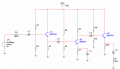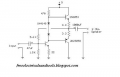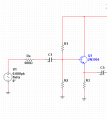# Designing a Multistage BJT Amplifier

Status
Not open for further replies.

#### ronsimpson

Joined Oct 7, 2019
1,156
Please give it a try.
We know the input and output are AC coupled. So draw capacitors at input and output. The input cap can be small because the impedance >20k. The output cap is large because the load is 600 ohms. Note it must pass 20hz. Do you know how to choose the capacitor?

I know you can not use a op-amp. But you can start out with a op-amp and get the gain of 50, the input capacitor and output capacitor parts working. Then go beck and design a simple 3 stage op-amp circuit using transistors.

There is a comment about 3 stages. Input, gain, output.
The output stage needs to pull almost to 0V and almost to 10V.

Do some part if this and come back and ask questions.

Thread Starter

#### ap30

Joined Aug 7, 2020
10
Please give it a try.
We know the input and output are AC coupled. So draw capacitors at input and output. The input cap can be small because the impedance >20k. The output cap is large because the load is 600 ohms. Note it must pass 20hz. Do you know how to choose the capacitor?

I know you can not use a op-amp. But you can start out with a op-amp and get the gain of 50, the input capacitor and output capacitor parts working. Then go beck and design a simple 3 stage op-amp circuit using transistors.

There is a comment about 3 stages. Input, gain, output.
The output stage needs to pull almost to 0V and almost to 10V.

Do some part if this and come back and ask questions.
So I've done this is the schematic i made, I know we have to go CC CE CC but im not sure what values i should make the resistors and capacitors.#### ronsimpson

Joined Oct 7, 2019
1,156
Good. Do you have "spice" so you can build the amplifier in your computer?
"three stages" I think you can use more than three transistors.
Q1 has a voltage gain of 1, Q3 has a voltage gain of 1, so all the gain must be in Q2. I think you need some gain in Q1.

Look up simple audio amplifiers on the internet. Here is an example of what you will find. They use power transistors in the output stage because they are driving 8 ohm load. You can use the 2n3904 & 2n3906 transistors because your load is 1k or 600 ohms. Take 470 to 4700 and 3.3 to 33.

This amp does not have enough gain. (only two stages) But it is a good place to learn from.Thread Starter

#### ap30

Joined Aug 7, 2020
10
Im using Multisim to build them. I just wanted to ask again but what values should I use for my capacitors. Thanks a lot.

#### ZCochran98

Joined Jul 24, 2018
131
Your capacitor choices should be made based on your bandwidth requirements. Keep in mind: the coupling capacitors (which you can choose, thankfully) typically set your low frequency cutoff, and the parasitic capacitors (the ones inherent in the transistors themselves - usually pretty small) are typically responsible for high-frequency cutoff. So think to yourself: how is a cutoff frequency related to capacitance? Does it go up or down as a capacitance is increased or decreased?

In the meantime, the resistor choices are much harder to do: you need to choose them to satisfy your current requirements, gain requirements, input/output impedance requirements, and assist with the low and high frequency cutoff point requirements.

I would recommend you focus first on the component values for the common-emitter stage first, then move onto the common-collector stages (as they're pretty simple, comparatively). Write up all the equations you know, fill in the blanks with values you know (like requirements or pre-guessed components), and proceed from there. At some point, you may just need to "just decide" a component value to go on - choose one based on previous experience or example.

Also keep in mind: you can cascade multiple common-emitter stages if necessary to get your gain up to the required point. Considering that it's a low gain of 50, it should be achievable with a single common-emitter circuit.

As a side comment, Multisim, thankfully, is a wonderful environment to tinker around with circuitry. Personally, I find it infinitely easier to use than LTSpice. Come up with a basic idea or design, then tweak values as necessary.

#### ronsimpson

Joined Oct 7, 2019
1,156
Did you study this in class?
input resistance no smaller than 20K
Supply=10V. 2N3904 current gain larger than 100.
Set Q1 base=2V (I just picked a number)
Just to keep the math simple R2=2k, R1=8k total = 10k. Do you see how that works?
R3 will change things a little. If R3=1k and the gain is 100 then the Base will look like 100k to ground. (Ask questions)
100k parallel with R2=2k. So the 100k will not change things much.
Do some math: what is the impedance at the input of the amp? Tell me how do you do this.How will you change the values of R1...R3 so you have a value larger than 20K. You can pick any number. 40k,100k, 25k...

Now to find the value of C1:
Some where you have a formula for F=1/(something)*(something)*R*C. Find that formula in your book.
F= must be smaller than 20hz. So pick a number. 10hz
R= the input impedance of the input state. (larger than 20k)
C=? That is the unknown

If you get really lost; run the simulation. Do only this one transistor circuit. (frequency sweep from 10hz to 100khz) See what happens. Change the value of C1 and see what happens to the frequency response at the low end.

#### MrAl

Joined Jun 17, 2014
8,062
So I've done this is the schematic i made, I know we have to go CC CE CC but im not sure what values i should make the resistors and capacitors.
View attachment 214230
Just a quick question for you...

Why do you have only one stage with gain when you have three transistors to work with?
The two voltage followers do not provide voltage gain and from the spec's you need at least 50 with no load and nearly that with load, so that leaves the entire gain of 50 tasked to that one transistor Q2.
A single transistor that has to handle a gain of 50 all by itself is going to produce more distortion as a byproduct than if two transistors share the gain If two transistors share the gain then each transistor stage only has to provide a gain of approximately 7, which is much much less than 50. That means less overall distortion. For two transistors that share gain equally the gain for each transistor is the square root of the entire gain. The square root of 50 is about 7.07 which is close to 7 or if you like you can use 7.1 to be sure.

So i would advise to design something that uses two of the transistors to share the gain.

Thread Starter

#### ap30

Joined Aug 7, 2020
10
Did you study this in class?

Supply=10V. 2N3904 current gain larger than 100.
Set Q1 base=2V (I just picked a number)
Just to keep the math simple R2=2k, R1=8k total = 10k. Do you see how that works?
R3 will change things a little. If R3=1k and the gain is 100 then the Base will look like 100k to ground. (Ask questions)
100k parallel with R2=2k. So the 100k will not change things much.
Do some math: what is the impedance at the input of the amp? Tell me how do you do this.
View attachment 214238
How will you change the values of R1...R3 so you have a value larger than 20K. You can pick any number. 40k,100k, 25k...

Now to find the value of C1:
Some where you have a formula for F=1/(something)*(something)*R*C. Find that formula in your book.
F= must be smaller than 20hz. So pick a number. 10hz
R= the input impedance of the input state. (larger than 20k)
C=? That is the unknown

If you get really lost; run the simulation. Do only this one transistor circuit. (frequency sweep from 10hz to 100khz) See what happens. Change the value of C1 and see what happens to the frequency response at the low end.
We went of the input resistance very briefly so I dont really remember it. The impedance at the input of the transistor would be R1//R2 correct? So we would have 1.6k. If you increase both R1 and R2 to 40k you would have 20k as the valuse so i can put anything higher.

Thread Starter

#### ap30

Joined Aug 7, 2020
10
Just a quick question for you...

Why do you have only one stage with gain when you have three transistors to work with?
The two voltage followers do not provide voltage gain and from the spec's you need at least 50 with no load and nearly that with load, so that leaves the entire gain of 50 tasked to that one transistor Q2.
A single transistor that has to handle a gain of 50 all by itself is going to produce more distortion as a byproduct than if two transistors share the gain If two transistors share the gain then each transistor stage only has to provide a gain of approximately 7, which is much much less than 50. That means less overall distortion. For two transistors that share gain equally the gain for each transistor is the square root of the entire gain. The square root of 50 is about 7.07 which is close to 7 or if you like you can use 7.1 to be sure.

So i would advise to design something that uses two of the transistors to share the gain.
This is the first time doing something like this so I am kind of lost. How would i go about making 2 transistors share the gain. Thanks#### MrAl

Joined Jun 17, 2014
8,062
This is the first time doing something like this so I am kind of lost. How would i go about making 2 transistors share the gain. ThanksOh, well do you know how to design a one transistor amplifier with a given gain?
That would be a prerequisite for designing a multi stage transistor amplifier.

Thread Starter

#### ap30

Joined Aug 7, 2020
10
If im only given the gain i would not know how to do it but if im given other parameters i might know how to do it. Also i have a question how do you get the quiescent current drawn from the power supply

#### sparky 1

Joined Nov 3, 2018
486
10uF will work.

If the project is exciting to you that is important. Many times defining the goals can go back and forth realizing the cost
The parameters come mostly when we define the goal. what is the input what is the output, stuff like that.
I like the three transistor 2N3904, 2N3906 It has been a classic. The 2N2905 2N3053 would be second choice.
Maybe that one will be interesting and informative. There is thread that covers it. It has a multisim, video :
https://forum.allaboutcircuits.com/threads/problem-with-designing-an-audio-amplifier.103470/

#### MrAl

Joined Jun 17, 2014
8,062
If im only given the gain i would not know how to do it but if im given other parameters i might know how to do it. Also i have a question how do you get the quiescent current drawn from the power supply
Hello again,

Are you using Spice or are you doing the calculations themselves for the circuit analysis?
I recommend both really.

Assuming a one transistor circuit amplifier to begin with...

In Spice, you would draw the circuit with some resistors and then use Spice to measure the current through the collector. We could talk about resistor values, but what kind of circuits have you designed in the past? That would help me to know where are you academically.

For manual calculations, you would write equations for the circuit and then try to solve for some resistor values. You could start with some simple assumptions like:
Gain=Rc/Re
Rc being the collector resistor, Re being an emitter resistor.
That's definitely not exact (and can be very far off once you add capacitors) but it will get you going at least.

It would be good if you described what kind of circuits you have analyzed in the past, even if you did not design them yourself.

Status
Not open for further replies.
Similar threads